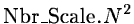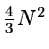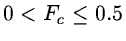Next: RECONS/WAVE Up: Commands Description Previous: Commands Description

### TRANSF/WAVE

TRANSF/WAVE Image Wavelet [Algo] [Nbr_Scale] [Fc]
This command creates a file which contains the wavelet transform. The suffixe of a wavelet transform file is .wave''. It is automatically added to the name passed to the command. Several algorithms are proposed:
1.
à trous algorithm with a linear scaling function. The wavelet function is the difference between two resolutions (see 14.4.3).
2.
à trous with a B3-spline scaling function (default value). The wavelet function is the difference between two resolutions (see 14.4.3).
3.
algorithm using the Fourier transform, without any reduction of the samples between two scales. The Fourier transform of the scaling function is a b3-spline and the wavelet function is the difference between two resolutions (14.4.5).
4.
pyramidal algorithm in the direct space, with a linear scaling function (see section 14.4.4).
5.
pyramidal algorithm in the direct space, with a b3-spline scaling function (see section 14.4.4).
6.
algorithm using the Fourier transform with a reduction of the samples between two scales. The Fourier transform of the scaling function is a b3-spline the wavelet function is the difference between two resolutions (14.4.5).
7.
algorithm using the Fourier transform with a reduction of the samples between two scales. The Fourier transform of the scaling function is a b3-spline. The wavelet function is the difference between the square of two resolutions (14.4.5).
8.
Mallat's Algorithm with biorthogonal filters (14.4.2).
The parameter Algo can be chosen between 1 and 8. If Algo is in {1,2,3}, the number of data of the wavelet transform is equal to the number of pixels multiplied by the number of scales (if the number of pixels of the image is N2, the number of wavelet coefficients is). Algorithms 4, 5, 6, and 7 are pyramidal (the number of wavelet coefficients is), and the 8th algorithm does not increase the number of data (the size of the wavelet transform is N2). Due to the discretisation and the undersampling, the properties of these algorithms are not the same. The 8th algorithm is more compact, but is not isotropic (see section 14.4.2). Algorithms 3, 6, and 7 compute the wavelet transform in the Fourier space (see section 14.4.5) and the undersampling respect Shannon's theorem. Pyramidal algorithms 4 and 5 compute the wavelet transform in the direct space, but need an interative reconstruction. Algorithms 1 and 2 are isotropic but increase the number of data. The 2D-discrete wavelet transform is not restricted the previous algorithms. Other algorithms exist (see for example Feauveau's one  which is not diadic). The interest of the wavelet transform is that it is a very flexible tool. We can adapt the transform to our problem. We prefer the 8th for image compression, 6 and 7 for image restoration, 2 for data analysis, etc.. The wavelet function can be derived too from the specific problem to resolve (see ).

The parameter Nbr_Scale specifies the number of scales to compute. The wavelet transform will containwavelet coefficients planes and one plane which will be the image at a very low resolution. The parameter Fc defines the cut-off frequency of the scaling function (). It is used only if the selected wavelet transform algorithm uses the FFT.Next: RECONS/WAVE Up: Commands Description Previous: Commands Description
Petra Nass
1999-06-15